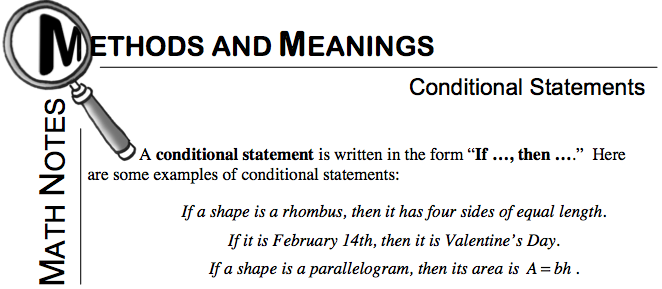### Home > GC > Chapter 3 > Lesson 3.2.1 > Problem3-50

3-50.

Consider the following arrow diagram: Homework Help ✎

Lines are parallel → alternate interior angles are equal.

1. Write this arrow diagram as a conditional (“If…, then…”) statement.

2. Write a similar conditional statement about corresponding angles, then write it as an arrow diagram.

Look at the Math Notes box from Lesson 2.2.3 for extra help.